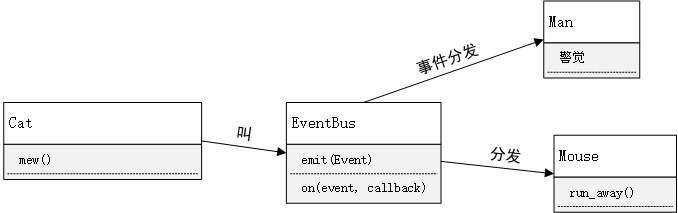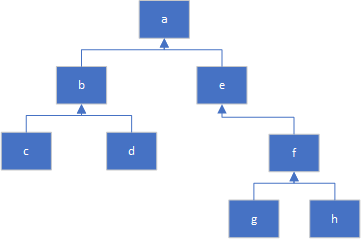# ████公司 C++ 笔试+面试回顾

2020/06/29 18:58

## 笔试部分

### 基础题

sizeof(a)返回的是数组大小，即400。我没注意数据类型是int，答成了100。实际上int的大小也不一定是4字节，我没记错的话按照标准文档的定义是不小于2字节，例如Win16 平台。现代32-bits/64-bits的体系结构基本能保证32-bits以上。

strlen(a)则是一个坑，按照题干中描述的int a的写法，数组a是未初始化的，数组内容依照标准规定是undefined behavior。strlen函数在遇到NUL的时候返回，这是典型的数组越界访问情形。而越界访问也是典型的undefined behavior。所以这题的正确答案应该是undefined behavior。

1. new (placement) type initializer
2. new type initializer

// 使用placement new必须引入这个标准头文件
#include <new>
#include <cassert>

struct Student {
int id;
char name;
};

int main() {
// example 1
// 常规 new 表达式
auto student = new Student;
// example 2
// placement new，在一片已经分配的内存上构造对象
auto mem = new char[sizeof(Student)];
auto student = new(mem) Student;
assert(static_cast<void*>(mem) == static_cast<void*>(student));
return 0;
}


placement new有很多细节可以在文档查阅到，放个链接在这里。cpp reference - new expression

## 分析题

#include <iostream>

using namespace std;

class A {
public:
A() {
cout << "A::A()" << endl;
init();
}

virtual ~A() {
cout << "A::~A()" << endl;
}

virtual void init() {
cout << "A::init()" << endl;
}

virtual void method() {
cout << "A::method()" << endl;
}
};

class B: public A {
public:
B(): A() {
cout << "B::B()" << endl;
}

~B() {
cout << "B::~B()" << endl;
}

void init() {
cout << "B::init()" << endl;
}

void method() {
cout << "B::method()" << endl;
}
};

int main() {
A* a = new B();
a->method();
delete a;
return 0;
}


B():A()显然调用了父类构造函数，按顺序应该是父类构造完毕再构造子类，在父类A::A()里调用了init();init是一个虚函数......所以调用的还是A::init。注意，在构造和析构函数中调用虚函数，调用的并不是派生类的覆盖函数，而是当前类中的最终覆盖函数。

A::A()
A::init()
B::B()


B::method()


B::~B()
A::~A()


1. 具有联动性，老鼠和主人的行为是被动的
2. 考虑扩展性，猫的叫声可能引发其他联动效应## 算法题#include <iostream>
#include <vector>

using namespace std;

int find_second(const vector<int>& container) {
int max=0;
int second=0;
for(const auto& i: container) {
if(i>max) {
second=max;
max=i;
}
if(i<max && i>second) {
second = i;
}
}

return second;
}

int main() {
cout << "second is " << find_second(vector<int> {1,2,3,4,5,6,7,9,10}) << endl;
}


## 面试部分2 评论
1 收藏
0